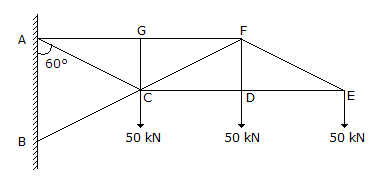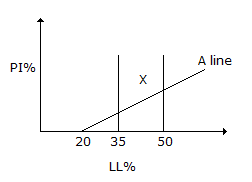# Civil Engineering - UPSC Civil Service Exam Questions

36.

The force in member FD in the given figure isA. 50 kN compression B. 50 kN tension C. 150 kN tension D. 100 kN compression

Explanation:

No answer description available for this question. Let us discuss.

37.

The ratio of the flexural strengths to two beams of square c. oss-section, the first beam being placed with its top and bottom sides horizontally and the second beam being placed with one diagonal horizontally, is

 A. 3 B. 1/3 C. 1/2 D. 2

Explanation:

No answer description available for this question. Let us discuss.

38.

The standard plasticity chart to classify fine grained soils is shown in the given figure. The area marked 'X' representsA. silt of low plasticity B. clay of high plasticity C. organic soil of medium plasticity D. clay of intermediate plasticity

Explanation:

No answer description available for this question. Let us discuss.

39.

Which one of the following statements is correct ? In the lime-soda process of water softening

 A. lime reduces carbonate hardness while soda removes non-carbonate hardness B. only carbonate hardness is removed C. lime reduces non-carbonate hardness while soda removes carbonate hardness D. Only non-carbonate hardness is removed.

Explanation:

No answer description available for this question. Let us discuss.

40.

In standard penetration test, the splitspoon sampler is penetrated into the soil stratum by giving blows from a drop weight whose weight (in kg) and free fall (in cm) are, respectively,

 A. 30 and 60 B. 60 and 30 C. 65 and 75 D. 75 and 65